• lstm时间序列预测代码
千次阅读 热门讨论
2022-04-22 16:53:38

大家好，今天和各位分享一下如何使用循环神经网络LSTM完成时间序列预测，本文是针对单个特征的预测，下一篇是对多个特征的预测。文末有完整代码

# 1. 导入工具包

这里使用GPU加速计算，加快网络的训练速度。

import tensorflow as tf
from tensorflow import keras
import numpy as np
import pandas as pd
import matplotlib.pyplot as plt
import warnings
warnings.filterwarnings('ignore')
# 调用GPU加速
gpus = tf.config.experimental.list_physical_devices(device_type='GPU')
for gpu in gpus:
tf.config.experimental.set_memory_growth(gpu, True)


# 2. 获取数据集

数据集有需要的自取：https://pan.baidu.com/s/1uWW7w1Ci04U3d8YFYPf3Cw  提取码：00qw

借助pandas库读取电量时间序列数据，两列特征数据，时间和电量

#（1）获取数据，按时间间隔1h记录的电力数据
filepath = 'energy.csv'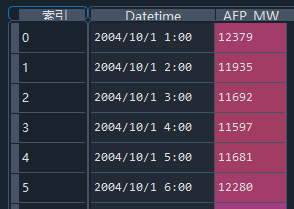# 3. 数据预处理

由于是基于时间序列的预测，将数据中的索引变成时间，取AFP电量特征列作为训练的特征

由于原始数据最大值和最小值之间相差较大，为了避免数据影响网络训练的稳定性，对训练用的特征数据进行标准化处理。

#（3）选择特征
temp = data['AEP_MW'] # 获取电力数据
temp.index = data['Datetime'] # 将索引改为时间序列
temp.plot()  # 绘图展示

#（4）对训练集预处理
temp_mean = temp[:train_num].mean()  # 均值
temp_std = temp[:train_num].std()  # 标准差
# 标准化
inputs_feature = (temp - temp_mean) / temp_std

绘制原始数据分布图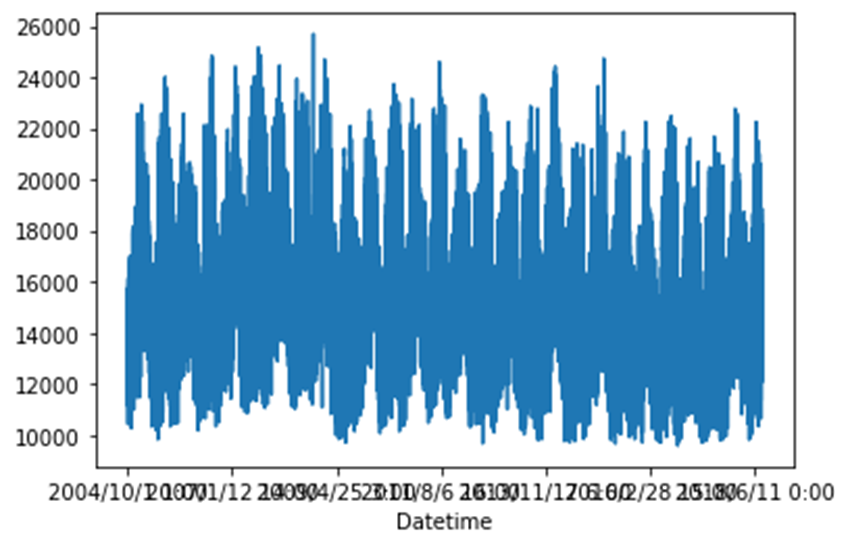# 4. 划分数据集

首先，需要通过时间序列滑动窗口选择特征值及其对应的标签值。比如对某一时间点预测，规定每20个特征值，预测得到一个标签值。由于只有一列特征数据，相当于，用前20个数据预测第21个数据。同理对某一时间片段预测，用第1到20个数据预测第21到30的电量。

#（2）构建时间序列采样函数
'''
dataset为输入的特征数据，选取用哪些特征
start_index 这么多数据选择从哪个开始，一般从0开始取序列
history_size表示时间窗口大小；若为20，代表从起始索引开始找20个样本当作x，下一个索引当作y
target_size表示需要预测的结果时窗口后的第几个时间点；0表示下一时间点的预测结果，取其当作标签；若为一个序列，预测一个序列的指标
indices=range(i, i+history_size) 代表窗口序列的索引，i表示每个窗口的起始位置，窗口中所有数据的索引
'''
def database(dataset, start_index, end_index, history_size, target_size):
data = []  # 存放特征值
labels = []  # 存放目标值

# 初始的取值片段[0:history_size]
start_index = start_index + history_size

# 如果不指定特征值终止索引，就取到最后一个分区前
if end_index is None:
end_index = len(dataset) - target_size

# 遍历整个电力数据，取出特征及其对应的预测目标
for i in range(start_index, end_index):
indices = range(i - history_size, i) # 窗口内的所有元素的索引
# 保存特征值和标签值
data.append(np.reshape(dataset[indices], (history_size, 1)))
labels.append(dataset[i+target_size]) # 预测未来几个片段的天气数据
# 返回数据集
return np.array(data), np.array(labels)

接下来就可以在原始数据集中划分训练集、验证集、测试集分别占比 90:9.8:0.2

# 取前90%个数据作为训练集
train_num = int(len(data) * 0.90)
# 90%-99.8%用于验证
val_num = int(len(data) * 0.998)
# 最后1%用于测试

#（5）划分训练集和验证集
# 窗口为20条数据，预测下一时刻气温
history_size = 20
target_size=0

# 训练集
x_train, y_train = database(inputs_feature.values, 0, train_num,
history_size, target_size)

# 验证集
x_val, y_val = database(inputs_feature.values, train_num, val_num,
history_size, target_size)

# 测试集
x_test, y_test = database(inputs_feature.values, val_num, None,
history_size, target_size)

# 查看数据信息
print('x_train.shape:', x_train.shape)  # x_train.shape: (109125, 20, 1)

# 5. 构造数据集

将划分好的numpy类型的训练集和验证集转换为tensor类型，用于网络训练。使用shuffle()函数打乱训练集数据，batch()函数指定每个step训练多少组数据。借助迭代器iter()使用next()函数从数据集中取出一个batch的数据用于验证。

#（6）构造tf数据集
# 训练集
train_ds = tf.data.Dataset.from_tensor_slices((x_train, y_train))
train_ds = train_ds.shuffle(10000).batch(128)
# 验证集
val_ds = tf.data.Dataset.from_tensor_slices((x_val, y_val))
val_ds = val_ds.batch(128)

# 查看数据信息
sample = next(iter(train_ds))
print('x_batch.shape:', sample.shape, 'y_batch.shape:', sample.shape)
print('input_shape:', sample.shape[-2:])
# x_batch.shape: (128, 20, 1) y_batch.shape: (128,)
# input_shape: (20, 1)


# 6. 模型构建

由于本案例数据量比较少，特征也只有一个，因此不需要使用复杂网络，使用一个LSTM层用于提取特征，一个全连接层用于输出预测结果。

# 构造输入层
inputs = keras.Input(shape=sample.shape[-2:])
# 搭建网络各层
x = keras.layers.LSTM(8)(inputs)
x = keras.layers.Activation('relu')(x)
outputs = keras.layers.Dense(1)(x)  # 输出结果是1个
# 构造模型
model = keras.Model(inputs, outputs)
# 查看模型结构
model.summary()

网络架构如下：

Layer (type)                 Output Shape              Param #
=================================================================
input_1 (InputLayer)         [(None, 20, 1)]           0
_________________________________________________________________
lstm_1 (LSTM)                (None, 8)                 320
_________________________________________________________________
activation_1 (Activation)    (None, 8)                 0
_________________________________________________________________
dense_1 (Dense)              (None, 1)                 9
=================================================================
Total params: 329
Trainable params: 329
Non-trainable params: 0

# 7. 网络训练

#（8）模型编译

model.compile(optimizer=opt, loss='mae')  # 平均误差损失

#（9）模型训练
epochs=20
history = model.fit(train_ds, epochs=epochs, validation_data=val_ds)


训练过程如下：

Epoch 1/20
853/853 [==============================] - 5s 5ms/step - loss: 0.4137 - val_loss: 0.0878
Epoch 2/20
853/853 [==============================] - 4s 5ms/step - loss: 0.0987 - val_loss: 0.0754
---------------------------------------------------
---------------------------------------------------
Epoch 19/20
853/853 [==============================] - 4s 5ms/step - loss: 0.0740 - val_loss: 0.0607
Epoch 20/20
853/853 [==============================] - 4s 4ms/step - loss: 0.0736 - val_loss: 0.0628

# 8. 查看训练信息

history变量中保存了训练过程的所有信息，我们绘制训练集损失和验证集损失曲线。

#（10）获取训练信息
history_dict = history.history  # 获取训练的数据字典
train_loss = history_dict['loss']  # 训练集损失
val_loss = history_dict['val_loss']  # 验证集损失

#（11）绘制训练损失和验证损失
plt.figure()
plt.plot(range(epochs), train_loss, label='train_loss')  # 训练集损失
plt.plot(range(epochs), val_loss, label='val_loss')  # 验证集损失
plt.legend()  # 显示标签
plt.xlabel('epochs')
plt.ylabel('loss')
plt.show()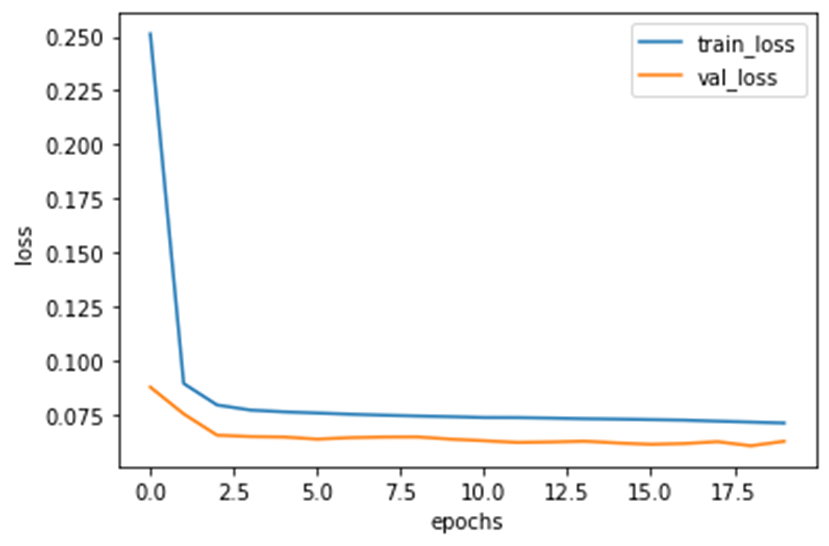# 9. 预测阶段

对先前划分好的测试集进行预测，model中保存了网络训练好了的权重，使用 predict() 函数预测特征 x_test 分别对应的电量 y_predict，真实值 y_test，绘图展示预测值和真实值的偏离程度。也可以计算预测值和真实值之间的方差或标准差等指标来表明预测的准确性。

#（12）预测
y_predict = model.predict(x_test)  # 对测试集的特征值进行预测

# x_test 等同于经过预处理后的 temp[val_num:-20].values
dates = temp[val_num:-20].index  # 获取时间索引

#（13）绘制预测结果和真实值对比图
fig = plt.figure(figsize=(10,5))
# 真实值
axes.plot(dates, y_test, 'bo', label='actual')
# 预测值，红色散点
axes.plot(dates, y_predict, 'ro', label='predict')
# 设置横坐标刻度
axes.set_xticks(dates[::30])
axes.set_xticklabels(dates[::30],rotation=45)

plt.legend()  # 注释
plt.grid()  # 网格
plt.show()

由于 x_test 对应原始数据中索引在 val_num 之后的特征信息，找到 x_test 中每个元素对应的时间 dates，作为x轴刻度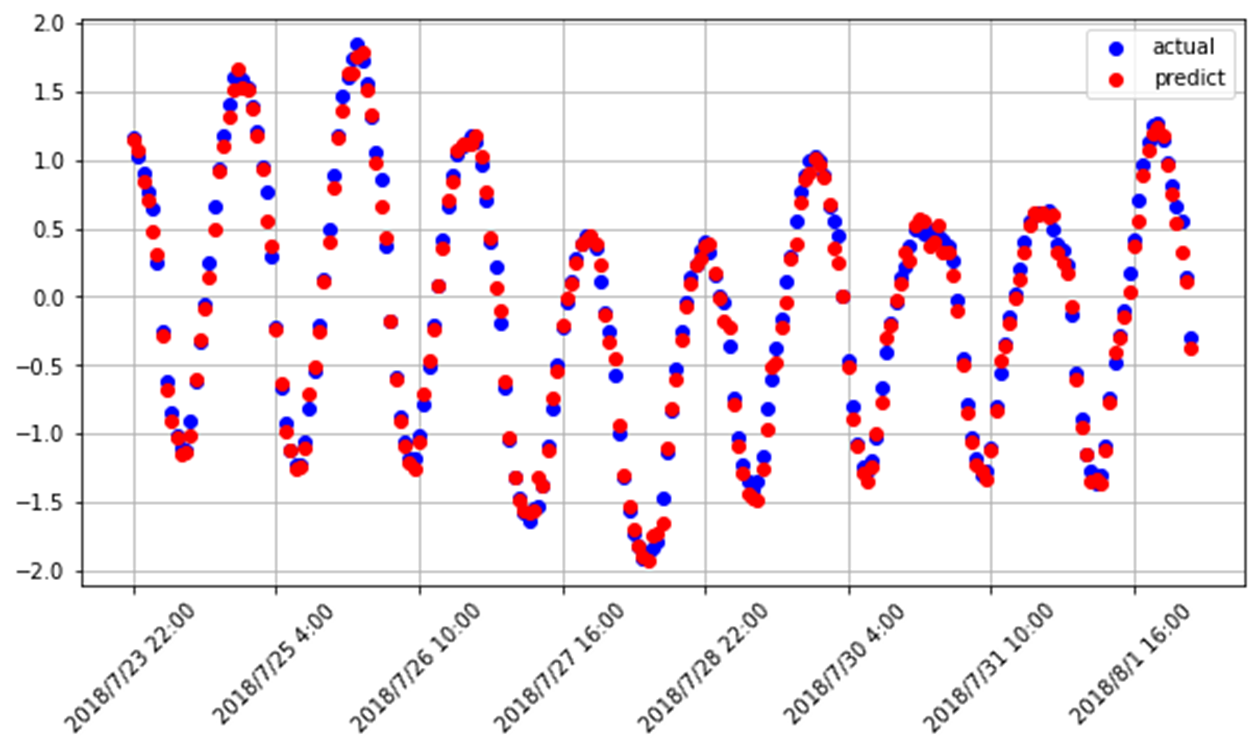python 神经网络 tensorflow 深度学习 LSTM
更多相关内容
• 时间序列预测问题中，建立LSTM模型，采用python语言代码实现
• 基于LSTM序列预测-机月流量预测项目代码，原始博客地址：https://panjinquan.blog.csdn.net/article/details/120918732
• 代码采用python语言写的一个LSTM时间序列预测销量LSTM 时间序列
• ## LSTM时间序列预测

千次阅读 2020-12-09 21:41:54
我们使用它来进行LSTM时间序列预测的实验。数据如图所示第一列为时间 第二列为数据编写代码头文件 import numpy import matplotlib.pyplot as plt from keras.models import Sequential from keras.layers impo

转载自：https://zhuanlan.zhihu.com/p/290683144

此数据是1949 到 1960 一共 12 年，每年 12 个月的航班乘客数据，一共 144 个数据，单位是 1000。我们使用它来进行LSTM时间序列预测的实验。
数据如图所示

第一列为时间 第二列为数据

## 编写代码

头文件

import numpy
import matplotlib.pyplot as plt
from keras.models import Sequential
from keras.layers import Dense
from keras.layers import LSTM
import  pandas as pd
import  os


加载数据
在这里我们设置时序数据的前65%为训练数据 后35%为测试数据

dataframe = pd.read_csv('./international-airline-passengers.csv', usecols=, engine='python', skipfooter=3)
dataset = dataframe.values
# 将整型变为float
dataset = dataset.astype('float32')
#归一化 在下一步会讲解
scaler = MinMaxScaler(feature_range=(0, 1))
dataset = scaler.fit_transform(dataset)

train_size = int(len(dataset) * 0.65)
trainlist = dataset[:train_size]
testlist = dataset[train_size:]


对数据进行处理
LSTM进行预测需要的是时序数据 根据前timestep步预测后面的数据
假定给一个数据集
{
A,B,C->D
B,C,D->E
C,D,E->F
D,E,F->G
E,F,G->H
}
这时timestep为3，即根据前三个的数据预测后一个数据的值
所以我们需要对数据进行转化
举一个简单的情况 假设一个list为[1,2,3,4,5],timestep = 2
我们转化之后要达到的效果是

train_Xtrain_Y

即依据前两个值预测下一个值

对数据进行归一化
LSTM可以不进行归一化的操作，但是这样会让训练模型的loss下降很慢。本教程如果不进行归一化，100次迭代后loss还是很高

#上面代码的片段讲解
scaler = MinMaxScaler(feature_range=(0, 1))
dataset = scaler.fit_transform(dataset)


对数据进行处理

def create_dataset(dataset, look_back):
#这里的look_back与timestep相同
dataX, dataY = [], []
for i in range(len(dataset)-look_back-1):
a = dataset[i:(i+look_back)]
dataX.append(a)
dataY.append(dataset[i + look_back])
return numpy.array(dataX),numpy.array(dataY)
#训练数据太少 look_back并不能过大
look_back = 1
trainX,trainY  = create_dataset(trainlist,look_back)
testX,testY = create_dataset(testlist,look_back)


LSTM模型
LSTM的输入为 [samples, timesteps, features]
这里的timesteps为步数，features为维度 这里我们的数据是1维的

trainX = numpy.reshape(trainX, (trainX.shape, trainX.shape, 1))
testX = numpy.reshape(testX, (testX.shape, testX.shape ,1 ))

# create and fit the LSTM network
model = Sequential()
model.fit(trainX, trainY, epochs=100, batch_size=1, verbose=2)
model.save(os.path.join("DATA","Test" + ".h5"))
# make predictions


进行预测

#model = load_model(os.path.join("DATA","Test" + ".h5"))
trainPredict = model.predict(trainX)
testPredict = model.predict(testX)

#反归一化
trainPredict = scaler.inverse_transform(trainPredict)
trainY = scaler.inverse_transform(trainY)
testPredict = scaler.inverse_transform(testPredict)
testY = scaler.inverse_transform(testY)


查看结果

plt.plot(trainY)
plt.plot(trainPredict[1:])
plt.show()
plt.plot(testY)
plt.plot(testPredict[1:])
plt.show()


这个时候我们的结果为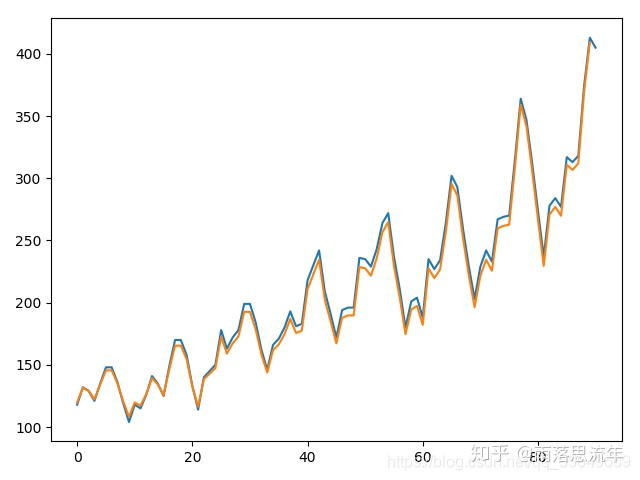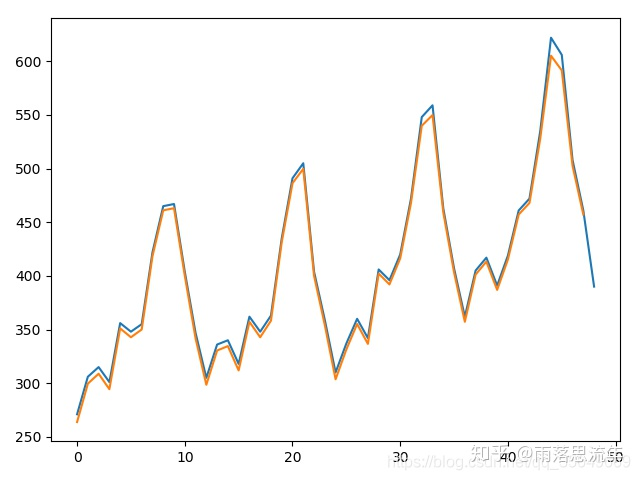展开全文• LSTM Time Series Prediction with Bayesian optimization.zipLSTM
• CNN结合BiLSTM实现数据预测python代码.zipmatlab
• ## 使用 LSTM 进行多变量时间序列预测

万次阅读 多人点赞 2022-01-11 10:13:30
使用 LSTM 进行端到端时间序列预测的完整代码和详细解释。 在本文中我们将使用深度学习方法 (LSTM) 执行多元时间序列预测。 我们先来了解两个主题—— 什么是时间序列分析？ 什么是 LSTM？ 时间序列分析：时间序列...

使用 LSTM 进行端到端时间序列预测的完整代码和详细解释。

在本文中我们将使用深度学习方法 (LSTM) 执行多元时间序列预测。

我们先来了解两个主题——

• 什么是时间序列分析？
• 什么是 LSTM？

时间序列分析：时间序列表示基于时间顺序的一系列数据。 它可以是秒、分钟、小时、天、周、月、年。 未来的数据将取决于它以前的值。

在现实世界的案例中，我们主要有两种类型的时间序列分析——

• 单变量时间序列
• 多元时间序列

对于单变量时间序列数据，我们将使用单列进行预测。正如我们所见，只有一列，因此即将到来的未来值将仅取决于它之前的值。

但是在多元时间序列数据的情况下，将有不同类型的特征值并且目标数据将依赖于这些特征。正如在图片中看到的，在多元变量中将有多个列来对目标值进行预测。 （上图中“count”为目标值）

在上面的数据中，count不仅取决于它以前的值，还取决于其他特征。因此，要预测即将到来的count值，我们必须考虑包括目标列在内的所有列来对目标值进行预测。

在执行多元时间序列分析时必须记住一件事，我们需要使用多个特征预测当前的目标，让我们通过一个例子来理解 -

在训练时，如果我们使用 5 列 [feature1, feature2, feature3, feature4, target] 来训练模型，我们需要为即将到来的预测日提供 4 列 [feature1, feature2, feature3, feature4]。

LSTM

本文中不打算详细讨论LSTM。所以只提供一些简单的描述，如果你对LSTM没有太多的了解，可以参考我们以前发布的文章。

LSTM基本上是一个循环神经网络，能够处理长期依赖关系。

假设你在看一部电影。所以当电影中发生任何情况时，你都已经知道之前发生了什么，并且可以理解因为过去发生的事情所以才会有新的情况发生。RNN也是以同样的方式工作，它们记住过去的信息并使用它来处理当前的输入。RNN的问题是，由于渐变消失，它们不能记住长期依赖关系。因此为了避免长期依赖问题设计了lstm。

现在我们讨论了时间序列预测和LSTM理论部分。让我们开始编码。

让我们首先导入进行预测所需的库

import numpy as np
import pandas as pd
from matplotlib import pyplot as plt
from tensorflow.keras.models import Sequential
from tensorflow.keras.layers import LSTM
from tensorflow.keras.layers import Dense, Dropout
from sklearn.preprocessing import MinMaxScaler
from keras.wrappers.scikit_learn import KerasRegressor
from sklearn.model_selection import GridSearchCV


加载数据，并检查输出-

df=pd.read_csv("train.csv",parse_dates=["Date"],index_col=)df.tail()现在让我们花点时间看看数据：csv文件中包含了谷歌从2001-01-25到2021-09-29的股票数据，数据是按照天数频率的。

[如果您愿意，您可以将频率转换为“B”[工作日]或“D”，因为我们不会使用日期，我只是保持它的现状。]

这里我们试图预测“Open”列的未来值，因此“Open”是这里的目标列

让我们看一下数据的形状

df.shape
(5203,5)


现在让我们进行训练测试拆分。这里我们不能打乱数据，因为在时间序列中必须是顺序的。

test_split=round(len(df)*0.20)
df_for_training=df[:-1041]
df_for_testing=df[-1041:]
print(df_for_training.shape)
print(df_for_testing.shape)

(4162, 5)
(1041, 5)


可以注意到数据范围非常大，并且它们没有在相同的范围内缩放，因此为了避免预测错误，让我们先使用MinMaxScaler缩放数据。(也可以使用StandardScaler)

scaler = MinMaxScaler(feature_range=(0,1))
df_for_training_scaled = scaler.fit_transform(df_for_training)
df_for_testing_scaled=scaler.transform(df_for_testing)
df_for_training_scaled将数据拆分为X和Y，这是最重要的部分，正确阅读每一个步骤。

def createXY(dataset,n_past):
dataX = []
dataY = []
for i in range(n_past, len(dataset)):
dataX.append(dataset[i - n_past:i, 0:dataset.shape])
dataY.append(dataset[i,0])
return np.array(dataX),np.array(dataY)

trainX,trainY=createXY(df_for_training_scaled,30)
testX,testY=createXY(df_for_testing_scaled,30)


让我们看看上面的代码中做了什么：

N_past是我们在预测下一个目标值时将在过去查看的步骤数。

这里使用30，意味着将使用过去的30个值(包括目标列在内的所有特性)来预测第31个目标值。

因此，在trainX中我们会有所有的特征值，而在trainY中我们只有目标值。

让我们分解for循环的每一部分

对于训练，dataset = df_for_training_scaled, n_past=30

当i= 30:

从n_past开始的范围是30，所以第一次数据范围将是-[30 - 30,30,0:5] 相当于 [0:30,0:5]

因此在dataX列表中，df_for_training_scaled[0:30,0:5]数组将第一次出现。

现在, dataY.append(df_for_training_scaled[i,0])

i = 30，所以它将只取第30行开始的open(因为在预测中，我们只需要open列，所以列范围仅为0，表示open列)。

第一次在dataY列表中存储df_for_training_scaled[30,0]值。

所以包含5列的前30行存储在dataX中，只有open列的第31行存储在dataY中。然后我们将dataX和dataY列表转换为数组，它们以数组格式在LSTM中进行训练。

我们来看看形状。

print("trainX Shape-- ",trainX.shape)
print("trainY Shape-- ",trainY.shape)

(4132, 30, 5)
(4132,)

print("testX Shape-- ",testX.shape)
print("testY Shape-- ",testY.shape)

(1011, 30, 5)
(1011,)


4132 是 trainX 中可用的数组总数，每个数组共有 30 行和 5 列， 在每个数组的 trainY 中，我们都有下一个目标值来训练模型。

让我们看一下包含来自 trainX 的 (30,5) 数据的数组之一 和 trainX 数组的 trainY 值

print("trainX-- \n",trainX)
print("trainY-- ",trainY)如果查看 trainX 值，会发现到它与 trainX 中的数据相同（第一列除外），因为我们将看到前 30 个来预测第 31 列，在第一次预测之后它会自动移动 到第 2 列并取下一个 30 值来预测下一个目标值。

让我们用一种简单的格式来解释这一切——

trainX — — →trainY

[0 : 30,0:5] → [30,0]

[1:31, 0:5] → [31,0]

[2:32,0:5] →[32,0]


像这样，每个数据都将保存在 trainX 和 trainY 中

现在让我们训练模型，我使用 girdsearchCV 进行一些超参数调整以找到基础模型。

def build_model(optimizer):
grid_model = Sequential()

grid_model.compile(loss = 'mse',optimizer = optimizer)
return grid_modelgrid_model = KerasRegressor(build_fn=build_model,verbose=1,validation_data=(testX,testY))

parameters = {'batch_size' : [16,20],
'epochs' : [8,10],

grid_search  = GridSearchCV(estimator = grid_model,
param_grid = parameters,
cv = 2)


如果你想为你的模型做更多的超参数调整，也可以添加更多的层。 但是如果数据集非常大建议增加 LSTM 模型中的时期和单位。

在第一个 LSTM 层中看到输入形状为 (30,5)。 它来自 trainX 形状。 (trainX.shape,trainX.shape) → (30,5)

现在让我们将模型拟合到 trainX 和 trainY 数据中。

grid_search = grid_search.fit(trainX,trainY)


由于进行了超参数搜索，所以这将需要一些时间来运行。

你可以看到损失会像这样减少——现在让我们检查模型的最佳参数。

grid_search.best_params_

{‘batch_size’: 20, ‘epochs’: 10, ‘optimizer’: ‘adam’}


将最佳模型保存在 my_model 变量中。

my_model=grid_search.best_estimator_.model


现在可以用测试数据集测试模型。

prediction=my_model.predict(testX)
print("prediction\n", prediction)
print("\nPrediction Shape-",prediction.shape)testY 和 prediction 的长度是一样的。 现在可以将 testY 与预测进行比较。

但是我们一开始就对数据进行了缩放，所以首先我们必须做一些逆缩放过程。

scaler.inverse_transform(prediction)报错了，这是因为在缩放数据时，我们每行有 5 列，现在我们只有 1 列是目标列。

所以我们必须改变形状来使用 inverse_transform

prediction_copies_array = np.repeat(prediction,5, axis=-1)5 列值是相似的，它只是将单个预测列复制了 4 次。所以现在我们有 5 列相同的值 。

prediction_copies_array.shape
(1011,5)


这样就可以使用 inverse_transform 函数。

pred=scaler.inverse_transform(np.reshape(prediction_copies_array,(len(prediction),5)))[:,0]


但是逆变换后的第一列是我们需要的，所以我们在最后使用了 → [:,0]。

现在将这个 pred 值与 testY 进行比较，但是 testY 也是按比例缩放的，也需要使用与上述相同的代码进行逆变换。

original_copies_array = np.repeat(testY,5, axis=-1)
original=scaler.inverse_transform(np.reshape(original_copies_array,(len(testY),5)))[:,0]


现在让我们看一下预测值和原始值 →

print("Pred Values-- " ,pred)
print("\nOriginal Values-- " ,original)最后绘制一个图来对比我们的 pred 和原始数据。

plt.plot(original, color = 'red', label = 'Real Stock Price')
plt.plot(pred, color = 'blue', label = 'Predicted Stock Price')
plt.title('Stock Price Prediction')
plt.xlabel('Time')
plt.legend()
plt.show()看样子还不错，到目前为止，我们训练了模型并用测试值检查了该模型。 现在让我们预测一些未来值。

从主 df 数据集中获取我们在开始时加载的最后 30 个值[为什么是 30？ 因为这是我们想要的过去值的数量，来预测第 31 个值]

df_30_days_past=df.iloc[-30:,:]
df_30_days_past.tail()可以看到有包括目标列（“Open”）在内的所有列。现在让我们预测未来的 30 个值。

在多元时间序列预测中，需要通过使用不同的特征来预测单列，所以在进行预测时我们需要使用特征值（目标列除外）来进行即将到来的预测。

df_30_days_future=pd.read_csv("test.csv",parse_dates=["Date"],index_col=)
df_30_days_future剔除“Open”列后，使用模型进行预测之前还需要做以下的操作：

缩放数据，因为删除了‘Open’列，在缩放它之前，添加一个所有值都为“0”的Open列。

缩放后，将未来数据中的“Open”列值替换为“nan”

现在附加 30 天旧值和 30 天新值（其中最后 30 个“打开”值是 nan）

df_30_days_future["Open"]=0
old_scaled_array=scaler.transform(df_30_days_past)
new_scaled_array=scaler.transform(df_30_days_future)
new_scaled_df=pd.DataFrame(new_scaled_array)
new_scaled_df.iloc[:,0]=np.nan
full_df=pd.concat([pd.DataFrame(old_scaled_array),new_scaled_df]).reset_index().drop(["index"],axis=1)


full_df 形状是 (60,5)，最后第一列有 30 个 nan 值。

要进行预测必须再次使用 for 循环，我们在拆分 trainX 和 trainY 中的数据时所做的。 但是这次我们只有 X，没有 Y 值

full_df_scaled_array=full_df.values
all_data=[]
time_step=30
for i in range(time_step,len(full_df_scaled_array)):
data_x=[]
data_x.append(
full_df_scaled_array[i-time_step :i , 0:full_df_scaled_array.shape])
data_x=np.array(data_x)
prediction=my_model.predict(data_x)
all_data.append(prediction)
full_df.iloc[i,0]=prediction


对于第一个预测，有之前的 30 个值，当 for 循环第一次运行时它会检查前 30 个值并预测第 31 个“Open”数据。

当第二个 for 循环将尝试运行时，它将跳过第一行并尝试获取下 30 个值 [1:31] 。这里会报错错误因为Open列最后一行是 “nan”，所以需要每次都用预测替换“nan”。

最后还需要对预测进行逆变换→

new_array=np.array(all_data)
new_array=new_array.reshape(-1,1)
prediction_copies_array = np.repeat(new_array,5, axis=-1)
y_pred_future_30_days = scaler.inverse_transform(np.reshape(prediction_copies_array,(len(new_array),5)))[:,0]
print(y_pred_future_30_days)这样一个完整的流程就已经跑通了。如果你想看完整的代码，可以在这里查看：

https://www.overfit.cn/post/1a36216705f2441b80fca567ea61e365

作者：Sksujanislam

展开全文lstm 深度学习 神经网络 机器学习 时间序列预测
• 长短时记忆网络（ Long short-term memory，LSTM ）是一种循环神经网络 (Recurrent neural network, RNN)的特殊变体。

目录

模型原理

模型实现

导入所需要的库

设置随机数种子

导入数据集

打印前五行数据进行查看

数据处理

归一化处理

查看归一化处理后的数据

将时间序列转换为监督学习问题

打印数据前五行

划分训练集和测试集

查看划分后的数据维度

搭建LSTM模型

得到损失图

模型预测

画图展示

得到预测图像

回归评价指标

# 模型原理

长短时记忆网络（ Long short-term memory，LSTM ）是一种循环神经网络 (Recurrent neural network, RNN)的特殊变体，具有“门”结构，通过门单元的逻辑控制决定数据是否更新或是选择丢弃，克服了 RNN 权重影响过大、容易产生梯度消失和爆炸的缺点，使网络可以更好、更快地收敛，能够有效提高预测精度。LSTM 拥有三个门， 分别为遗忘门、输入门、输出门，以此决定每一时刻信息记忆与遗忘。输入门决定有多少新的信息加入到细胞当中，遗忘门控制每一时刻信息是否会被遗忘，输出门决定每一时刻是否有信息输出。其基本结构如图所示。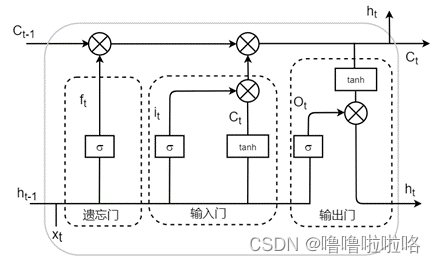公式如下：

（1）遗忘门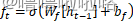（2）输入门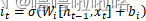（3）单元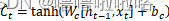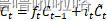（4）输出门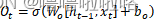（5）最终输出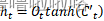# 模型实现

## 导入所需要的库

import matplotlib.pyplot as plt
from pandas import DataFrame
from pandas import concat
from sklearn.preprocessing import MinMaxScaler
from tensorflow.keras.models import Sequential
from tensorflow.keras.layers import LSTM,Dense,Dropout
from numpy import concatenate
from sklearn.metrics import mean_squared_error,mean_absolute_error,r2_score
from math import sqrt


## 设置随机数种子

import tensorflow as tf
tf.random.set_seed(2)

## 导入数据集

qy_data=read_csv(r'C:\Users\HUAWEI\Desktop\abc.csv',parse_dates=['num'],index_col='num')
qy_data.index.name='num' #选定索引列

### 打印前五行数据进行查看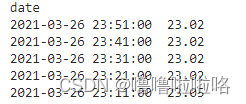## 数据处理

# 获取DataFrame中的数据，形式为数组array形式
values = qy_data.values
# 确保所有数据为float类型
values = values.astype('float32')

## 归一化处理

使用MinMaxScaler缩放器，将全部数据都缩放到[0,1]之间，加快收敛。

scaler = MinMaxScaler(feature_range=(0, 1))
scaled = scaler.fit_transform(values)

### 查看归一化处理后的数据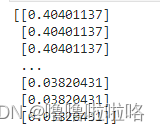## 将时间序列转换为监督学习问题

时间序列形式的数据转换为监督学习集的形式，例如：[,,,,]转换为[[0,10],[10,11],[11,12],[12,13],[13,14]]，即把前一个数作为输入，后一个数作为对应输出。

def series_to_supervised(data, n_in=1, n_out=1, dropnan=True):
n_vars = 1 if type(data) is list else data.shape
df = DataFrame(data)
cols, names = list(), list()
# input sequence (t-n, ... t-1)
for i in range(n_in, 0, -1):
cols.append(df.shift(i))
names += [('var%d(t-%d)' % (j + 1, i)) for j in range(n_vars)]
# forecast sequence (t, t+1, ... t+n)
for i in range(0, n_out):
cols.append(df.shift(-i))
if i == 0:
names += [('var%d(t)' % (j + 1)) for j in range(n_vars)]
else:
names += [('var%d(t+%d)' % (j + 1, i)) for j in range(n_vars)]
# put it all together
agg = concat(cols, axis=1)
agg.columns = names
# drop rows with NaN values
if dropnan:
agg.dropna(inplace=True)
return agg

reframed = series_to_supervised(scaled, 2, 1)

### 打印数据前五行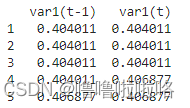## 划分训练集和测试集

# 划分训练集和测试集
values = reframed.values
trainNum = int(len(values) * 0.7)
train = values[:trainNum,:]
test = values[trainNum:, :]

### 查看划分后的数据维度

print(train_X.shape, train_y.shape)
print(test_X.shape, test_y.shape)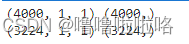## 搭建LSTM模型

初始化LSTM模型，设置神经元核心的个数，迭代次数，优化器等等

model = Sequential()
history = model.fit(train_X, train_y, epochs=95, batch_size=2, validation_data=(test_X, test_y), verbose=2,shuffle=False)

### 得到损失图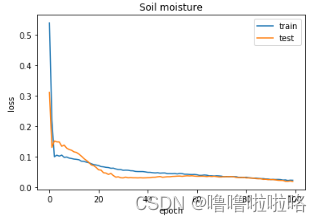​​​​​​​

## 模型预测

y_predict = model.predict(test_X)
test_X = test_X.reshape((test_X.shape, test_X.shape))

## 画图展示

plt.figure(figsize=(10,8),dpi=150)
plt.plot(inv_y,color='red',label='Original')
plt.plot(inv_y_predict,color='green',label='Predict')
plt.xlabel('the number of test data')
plt.ylabel('Soil moisture')
plt.legend()
plt.show()

### 得到预测图像

将测试集的y值和预测值绘制在同一张图表中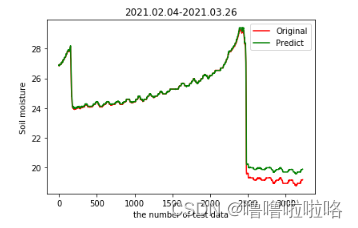## 回归评价指标

# calculate MSE 均方误差
mse=mean_squared_error(inv_y,inv_y_predict)
# calculate RMSE 均方根误差
rmse = sqrt(mean_squared_error(inv_y, inv_y_predict))
#calculate MAE 平均绝对误差
mae=mean_absolute_error(inv_y,inv_y_predict)
#calculate R square
r_square=r2_score(inv_y,inv_y_predict)
print('均方误差MSE: %.6f' % mse)
print('均方根误差RMSE: %.6f' % rmse)
print('平均绝对误差MAE: %.6f' % mae)
print('R_square: %.6f' % r_square)

展开全文lstm 深度学习
• ## pytorch LSTM时间序列预测

千次阅读 热门讨论 2021-12-02 11:28:49pytorch lstm python
• GA-LSTM 遗传算法优化的lstm 预测代码 python实现 直接就可以运行 GA.PY 具体可以通过lstm.py的load数据进行修改
• matlab,代码详细说明，自己出的，完全可运行使用
• ## LSTM时间序列预测

千次阅读 2021-05-23 09:19:36
LSTM时间序列预测（单维，多步预测一步） 文章目录LSTM时间序列预测（单维，多步预测一步）前言使用步骤1.引入库2.设置一下，画图时可以显示汉字3.设置监督学习格式3.读取数据4.缩放数据：归一化5.划分训练集和测试...机器学习 python lstm
• TensorFlow框架下的LSTM时间序列预测【简易】tensorflow lstm python
• 主要介绍了Python中利用LSTM模型进行时间序列预测分析的实现，文中通过示例代码介绍的非常详细，对大家的学习或者工作具有一定的参考学习价值，需要的朋友们下面随着小编来一起学习学习吧
• 数据是单维一例的，基于时间节点的预测，matlab出图，程序包含LSTM程序单独运行、SSA-LSTM程序运行、两种程序对比。前70%训练，后面30%预测，大约有2000个数据，代码有详细说明，可供调整学习。
• 时序预测 | MATLAB实现Bayes（贝叶斯）优化LSTM(长短期记忆神经网络)时间序列预测（完整源码和数据） 数据为一维时序列数据,运行环境MATLAB2018b及以上。
• tensorflow下用LSTM网络进行时间序列预测，实时多变量预测以及对于未来数据的单变量预测。
• LSTM时间序列预测方面的应用非常广，但有相当一部分没有考虑使用多长的数据预测下一个，类似AR模型中的阶数P。我基于matlab2021版编写了用LSTM模型实现多步预测时间序列的程序代码，可以自己调整使用的数据“阶数...
• 完整代码已上传我的资源：【LSTM时间序列数据】基于matlab LSTM时间序列数据预测【含Matlab源码 1949期】获取代码方式2： 通过订阅紫极神光博客付费专栏，凭支付凭证，私信博主，可获得此代码。备注： 订阅紫极神光...matlab lstm 开发语言
• # 代码功能: LS-TM 循环神经网络，预测时间序列---------------------------- # 第1步: 处理原始数据集, 归一化，制作X_train、Y_train、X_test、Y_test # 第2步: 训练LS-TM网络, epoch=300 ...# 第3步: 时间序列预测
• LSTM(Long Short-Term Memory)是长短期记忆网络，是一种时间递归神经网络，适合于处理和预测时间序列中间隔和延迟相对较长的重要事件。
• 时序预测 | MATLAB实现BiLSTM时间序列预测 目录时序预测 | MATLAB实现BiLSTM时间序列预测基本介绍程序设计单层网络多层网络参考资料致谢 基本介绍 双向 LSTM 或 biLSTM 是一种序列处理模型，由两个 LSTM 组成：一个...时间序列 深度学习 BiLSTM 多层网络
• 2 部分代码 clc; clear; close all; %% ---------------------------- init Variabels ---------------------------- opt.Delays = 1:30; opt.dataPreprocessMode = 'Data Standardization'; % 'None' 'Data ...matlab lstm 机器学习
• matlab出图，运行保障，代码有详细说明，适用初学者。
• 一、贝叶斯网络及LSTM简介 1 贝叶斯网络 贝叶斯网络(Bayesian network)，又称信念网络(Belief Network)，或有向无环图模型(directed acyclic graphical model)，是一种概率图模型，于1985年由Judea Pearl首先提出。...matlab
• LSTM的控制流程：是在前向传播的过程中处理流经细胞的数据，不同之处在于 LSTM 中细胞的结构和运算有所变化。 这一系列运算操作使得 LSTM具有能选择保存信息或遗忘信息的功能。咋一看这些运算操作时可能有点复杂，...matlab lstm
• 目录LSTM神经网络LSTM原理LSTM数据下载LSTM程序设计导入Python包时序数据处理LSTM模块定义模型训练模型评估参数设定运行结果参考资料致谢 LSTM神经网络 长短期记忆网络——通常简称为“LSTM”——是一种特殊的 RNN...神经网络 深度学习 python 机器学习...